NEET  >  Case Based Type Questions: The Solid State

# Case Based Type Questions: The Solid State - Chemistry Class 12 - NEET

Question 1: Read the passage given below and answer the following questions:
The adjective, ‘crystalline’ when applied to solids, implies an ideal crystal in which the structural units, termed as unit cells, are repeated regularly and indefinitely in three dimensions in space. The unit cell, containing at least one molecule has definite orientation and shape defined by the translational vectors, a, b and c. The unit cell therefore has a definite volume, V that contains the atoms and molecules necessary for generating the crystal. Every crystal can be classified as a member of one of the seven possible crystal systems or crystal classes that are defined by the relationship between the individual dimensions, a, b and c of the unit cell and between the individual angles, α, β, and γ of the unit cell. The structure of the given crystal may be assigned to one of the 7 crystal systems, to one of the 14 Brevais lattices, and to one of the 230 space groups. This uniquely define the possible ways of rearranging atoms in a three-dimensional solid, Based on these observations, seven crystal systems were identified: triclinic, monoclinic, trigonal or rhombohedral, tetragonal, hexagonal, rhombic or orthorhombic and cubic.
The following questions are multiple choice questions. Choose the most appropriate answer:

The crystal system of a compound with unit cell dimensions, a = 0.387 nm, b = 0.387 nm and c = 0.504 nm and α = β = 90° and γ =120° is
(a) cubic
(b) hexagonal
(c) orthorhombic
(d) rhombohedral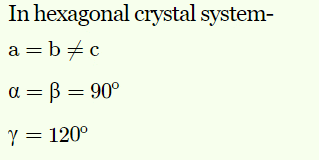In hexagonal crystal system-
a = b ≠ c
α = β = 90o
γ = 120o

Question 2: Read the passage given below and answer the following questions:
All real structures are three dimensional structures. They can be obtained by stacking two dimensional layers one above the other while placing the second square close packed layer above the first we follow the same rule that was followed when one row was placed adjacent to the other. The second layer is placed over the first layer such that the spheres of the upper layer are exactly above there of the first layer. In his arrangement spheres of both the layers are perfectly aligned horizontally as well as vertically. A metallic element crystallise into a lattice having a ABC ABC pattern and packing of spheres leaves out voids in the lattice.

What type of structure is formed by this arrangement?
(a) ccp
(b) hcp
(c) ccp/fcc
(d) none of the above

Question 3: Read the passage given below and answer the following questions:
The adjective, ‘crystalline’ when applied to solids, implies an ideal crystal in which the structural units, termed as unit cells, are repeated regularly and indefinitely in three dimensions in space. The unit cell, containing at least one molecule has definite orientation and shape defined by the translational vectors, a, b and c. The unit cell therefore has a definite volume, V that contains the atoms and molecules necessary for generating the crystal. Every crystal can be classified as a member of one of the seven possible crystal systems or crystal classes that are defined by the relationship between the individual dimensions, a, b and c of the unit cell and between the individual angles, α, β, and γ of the unit cell. The structure of the given crystal may be assigned to one of the 7 crystal systems, to one of the 14 Brevais lattices, and to one of the 230 space groups. This uniquely define the possible ways of rearranging atoms in a three-dimensional solid, Based on these observations, seven crystal systems were identified: triclinic, monoclinic, trigonal or rhombohedral, tetragonal, hexagonal, rhombic or orthorhombic and cubic.
The following questions are multiple choice questions. Choose the most appropriate answer:

The unit cell with the structure given below represents ________crystal system.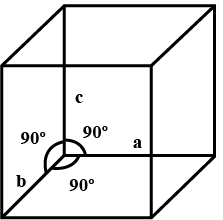(a) cubic

(b) orthorhombic

(c) tetragonal

(d) trigonal

If a = b = c and α = β = γ = 90o , the given structure will be a cubic crystal system.
Image I : a = b = c

all angles =90(Isometric) cubic
Image II : a ≠ b ≠ c
all angles = 90o
Orthorhombic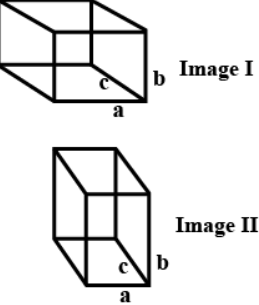Question 4: Read the passage given below and answer the following questions:
All real structures are three dimensional structures. They can be obtained by stacking two dimensional layers one above the other while placing the second square close packed layer above the first we follow the same rule that was followed when one row was placed adjacent to the other. The second layer is placed over the first layer such that the spheres of the upper layer are exactly above there of the first layer. In his arrangement spheres of both the layers are perfectly aligned horizontally as well as vertically. A metallic element crystallise into a lattice having a ABC ABC pattern and packing of spheres leaves out voids in the lattice.

Name the non-stoichiometric point defect responsible for colour in alkali metal halides.
(a) Frenkel defect
(b) Interstitial defect
(c) Schottky defect
(d) F-centres

Question 5: Read the passage given below and answer the following questions:
The adjective, ‘crystalline’ when applied to solids, implies an ideal crystal in which the structural units, termed as unit cells, are repeated regularly and indefinitely in three dimensions in space. The unit cell, containing at least one molecule has definite orientation and shape defined by the translational vectors, a, b and c. The unit cell therefore has a definite volume, V that contains the atoms and molecules necessary for generating the crystal. Every crystal can be classified as a member of one of the seven possible crystal systems or crystal classes that are defined by the relationship between the individual dimensions, a, b and c of the unit cell and between the individual angles, α, β, and γ of the unit cell. The structure of the given crystal may be assigned to one of the 7 crystal systems, to one of the 14 Brevais lattices, and to one of the 230 space groups. This uniquely define the possible ways of rearranging atoms in a three-dimensional solid, Based on these observations, seven crystal systems were identified: triclinic, monoclinic, trigonal or rhombohedral, tetragonal, hexagonal, rhombic or orthorhombic and cubic.
The following questions are multiple choice questions. Choose the most appropriate answer:

In a triclinic crystal

(a) a = b = c, α = β = γ ≠ 90°

(b) a ≠ b = c, α = β = γ = 90°

(c) a ≠ b ≠ c, α ≠ β ≠ γ ≠ 90°

(d) a ≠ b ≠ c, α = γ = 90°, β ≠ 90°

Question 6: Read the passage given below and answer the following questions:
All real structures are three dimensional structures. They can be obtained by stacking two dimensional layers one above the other while placing the second square close packed layer above the first we follow the same rule that was followed when one row was placed adjacent to the other. The second layer is placed over the first layer such that the spheres of the upper layer are exactly above there of the first layer. In his arrangement spheres of both the layers are perfectly aligned horizontally as well as vertically. A metallic element crystallise into a lattice having a ABC ABC pattern and packing of spheres leaves out voids in the lattice.

What is the total volume of atoms in a face centred cubic unit cell of a metal? (r is atomic radius).
(a) 16/3 πr3
(b) πr3
(c) 24/3 πr3
(d) 12/3 πr3

Number of atoms per unit cell in fcc = 4
∴ Total volume of atoms present in fcc unit cell
= 4 x (4/3)πr3 = 16/3πr3

Question 7: Read the passage given below and answer the following questions:
The adjective, ‘crystalline’ when applied to solids, implies an ideal crystal in which the structural units, termed as unit cells, are repeated regularly and indefinitely in three dimensions in space. The unit cell, containing at least one molecule has definite orientation and shape defined by the translational vectors, a, b and c. The unit cell therefore has a definite volume, V that contains the atoms and molecules necessary for generating the crystal. Every crystal can be classified as a member of one of the seven possible crystal systems or crystal classes that are defined by the relationship between the individual dimensions, a, b and c of the unit cell and between the individual angles, α, β, and γ of the unit cell. The structure of the given crystal may be assigned to one of the 7 crystal systems, to one of the 14 Brevais lattices, and to one of the 230 space groups. This uniquely define the possible ways of rearranging atoms in a three-dimensional solid, Based on these observations, seven crystal systems were identified: triclinic, monoclinic, trigonal or rhombohedral, tetragonal, hexagonal, rhombic or orthorhombic and cubic.
The following questions are multiple choice questions. Choose the most appropriate answer:

The unit cell with dimensions α = β = γ = 90°, a = b ≠ c is
(a) cubic
(b) triclinic
(c) hexagonal
(d) tetragonal

Or
An example of orthorhombic crystal system is
(a) SnO2
(b) KNO3
(c) ZnO
(d) K2Cr2O7

The unit cell with crystallographic dimensions a=b≠c and α=β=γ=90o is tetragonal.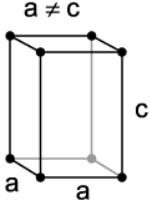OR

Question 8: Read the passage given below and answer the following questions:
All real structures are three dimensional structures. They can be obtained by stacking two dimensional layers one above the other while placing the second square close packed layer above the first we follow the same rule that was followed when one row was placed adjacent to the other. The second layer is placed over the first layer such that the spheres of the upper layer are exactly above there of the first layer. In his arrangement spheres of both the layers are perfectly aligned horizontally as well as vertically. A metallic element crystallise into a lattice having a ABC ABC pattern and packing of spheres leaves out voids in the lattice.

Which of the following statements not true for the amorphous and crystalline solids?
(a) Amorphous solids are isotropic and crystalline solids are anisotropic.
(b) Amorphous solids are short range order and crystalline solids are long range order.
(c) Amorphous solids melt at characteristic temperature while crystalline solids melt over a range of temperature.
(d) Amorphous solids have irregular shape and crystalline solids have a geometrical shape.

Or
Which of the following statements is not true for unit cell?
(a) Each cubic unit cell has 8 atoms on its corners the total number of atoms in one unit cell is 1.
(b) A un it cell is characterized by its dimensions along the three edges a, b, c.
(c) Each body centred cube cell has 2 atoms in one unit cell.
(d) Each face centred cubic cell contains only one constituent particle present at the centre of each face.

Crystalline solids melt at characteristic temperature while amorphous solids melt over a range of temperature.

Or

Each fcc unit cell contains one constituent particle present at the centre of each face, besides the ones that are at its corners.

Question 9: Read the passage given below and answer the following questions:
In ideally ionic structures, the coordination numbers of the ions are determined by electrostatic considerations. Cations surround themselves with as many anions as possible and vice versa. This maximizes the attractions between neigh-bouring ions of opposite charge and hence maximizes the lattice energy of the crystal. This requirement led to the formulation of the radius ratio rule for ionic structures in which the ions and the structure adopted for a particular compound depend on the relative sizes of the ions. Thus, for the stable ionic crystalline structures, there is definite radius ratio limit for a cation to fit perfectly in the lattice of anions, called radius ratio rule. This depends upon the ratio of radii of two types of ions, r+/r.
This ratio for coordination numbers 3, 4, 6 and 8 are respectively 0.155 – 0.225, 0.225 -0.414, 0.414 -0.732 and 0.732 – 1.000. The coordination number of ionic solids also depends upon temperature and pressure. On applying high pressure, coordination number increases. On the other hand, on applying high temperature, it decreases.
The following questions are multiple choice questions. Choose the most appropriate answer:

The ionic radii of K+, Rb+ and Br are 137, 148 and 195 pm. The coordination number of cation in RbBr and KBr structures are respectively
(a) 8,6
(b) 6,4
(c) 6,8
(d) 4,6
OR
For a coordination number 4, the maximum limiting radius ratio is
(a) 0.414
(b) 0.732
(c) 0.225
(d) 0.155

Or

Question 10: Study the diagram given below and answer the following questions: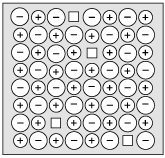In these questions, a statement of Assertion followed by a statement of Reason is given. Choose the correct answer out of the following choices.

Assertion (A): The diagram shows Schottky defect.

Reason (R): Schottky defect occurs in ionic solids.
(A) Both A and R are true and R is the correct explanation of A
(B) Both A and R are true but R is NOT the correct explanation of A
(C) A is true but R is false
(D) A is false and R is True

The diagram shows Schottky defect as it has equal number of cationic and anionic vacancies.

The document Case Based Type Questions: The Solid State | Chemistry Class 12 - NEET is a part of the NEET Course Chemistry Class 12.
All you need of NEET at this link: NEET

## FAQs on Case Based Type Questions: The Solid State - Chemistry Class 12 - NEET

 1. What is the definition of the solid state in NEET?Ans. The solid state, as defined in NEET, refers to the state of matter where particles are closely packed and have a fixed position. It is characterized by the strong intermolecular forces and the absence of free or mobile particles.
 2. How are solids different from liquids and gases in the NEET syllabus?Ans. Solids, in the NEET syllabus, differ from liquids and gases in terms of the arrangement and motion of particles. While solids have a fixed shape and volume with particles tightly packed, liquids have a fixed volume but no fixed shape, and gases have neither a fixed shape nor volume.
 3. What are some examples of crystalline solids mentioned in the NEET syllabus?Ans. Crystalline solids mentioned in the NEET syllabus include common substances like table salt (NaCl), diamond, quartz, and copper. These solids have a regular geometric arrangement of particles, forming well-defined crystal structures.
 4. Can you explain the concept of a unit cell in the context of the solid state?Ans. In the solid state, a unit cell refers to the smallest repeating unit of a crystal lattice. It represents the arrangement of atoms or ions that are repeated in three dimensions to form a crystal. The unit cell's dimensions and shape determine the overall crystal structure.
 5. What are the factors that affect the conductivity of solids, as per the NEET syllabus?Ans. The factors that affect the conductivity of solids, as per the NEET syllabus, include the nature of the material, temperature, and the presence of impurities. Metallic solids generally exhibit high conductivity due to the presence of delocalized electrons, while ionic and covalent solids may have lower conductivity. Temperature changes and impurities can also influence conductivity.

## Chemistry Class 12

154 videos|396 docs|261 tests

## Chemistry Class 12

154 videos|396 docs|261 tests

### How to Prepare for NEET

Read our guide to prepare for NEET which is created by Toppers & the best Teachers
Signup to see your scores go up within 7 days! Learn & Practice with 1000+ FREE Notes, Videos & Tests.
10M+ students study on EduRev
Track your progress, build streaks, highlight & save important lessons and more!(Scan QR code)
Related Searches

,

,

,

,

,

,

,

,

,

,

,

,

,

,

,

,

,

,

,

,

,

;